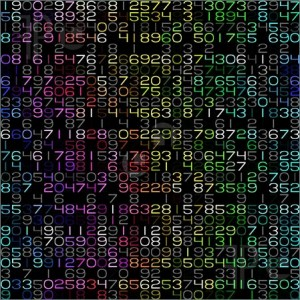# Odd Weird NumbersCommon intuition is a clandestine notion hidden behind the wall of mathematical formalism.  Elaborate systems that dispose of specification in favor of complete abstraction are merely derived from basic understanding.   Axiomatic truths build elegant mathematical structures layered by bricks of minor principles.  In example, by using only three postulates the entire theory of Calculus can be constructed.

Oftentimes, mathematical axioms are simply self-evident truths that are drawn from a set of numbers.     Number theorists, in particular, have found that numbers themselves exhibit their own special character.    Indeed, in the field of Number Theory, numbers can be abundant, semiperfect, and weird.   In fact, these strangely named numbers define a major unsolved problem.

An abundant number is defined as a number in which the sum of its proper divisors is greater than the number itself.    Take the number 24 for example.  Since {1,2,3,4,6,8,12} is the set of proper divisors ( all of the divisors except 24) of 24 and  1 + 2 + 3 + 4 + 6 + 8 + 12 = 36,  24 is an abundant number.   If a natural number is the sum of all or some of its proper divisors, then it is a semiperfect number.    Consequently, by inspection, a perfect number is a semiperfect number that is the sum of all of its proper divisors.

Considering these definitions, a weird number can be defined.  Weird numbers are natural numbers that are abundant but not semiperfect.    For example, 70 is the lowest weird number, because its set of proper divisors {1,2,5,7,10,14,35} sum to 74, but no subset of its set of proper divisors sum to the number 70, and 70 is the smallest number to meet such conditions.    The first few weird numbers are 70, 836, 4030, and 5830 and so on.

In this small sampling of weird numbers, one can see that they are all even.  Thus, the following question could be asked: Do any odd weird numbers exist?   This is an easy question to ask, but far from easy to answer.  However, other basic properties can be described with more ease.  For instance, mathematicians have proven that there are infinitely many weird numbers.  Also, if an odd weird number does exist, then it must be greater than$4 X 10^9$.

Therefore, it is unlikely that pure computation alone will answer the question.   Perhaps a solution is bound behind the wall of mathematical formalism.    However, the answer could emerge from behind the bricks into the light of common understanding.1.Jenaro Tomaszewski says: